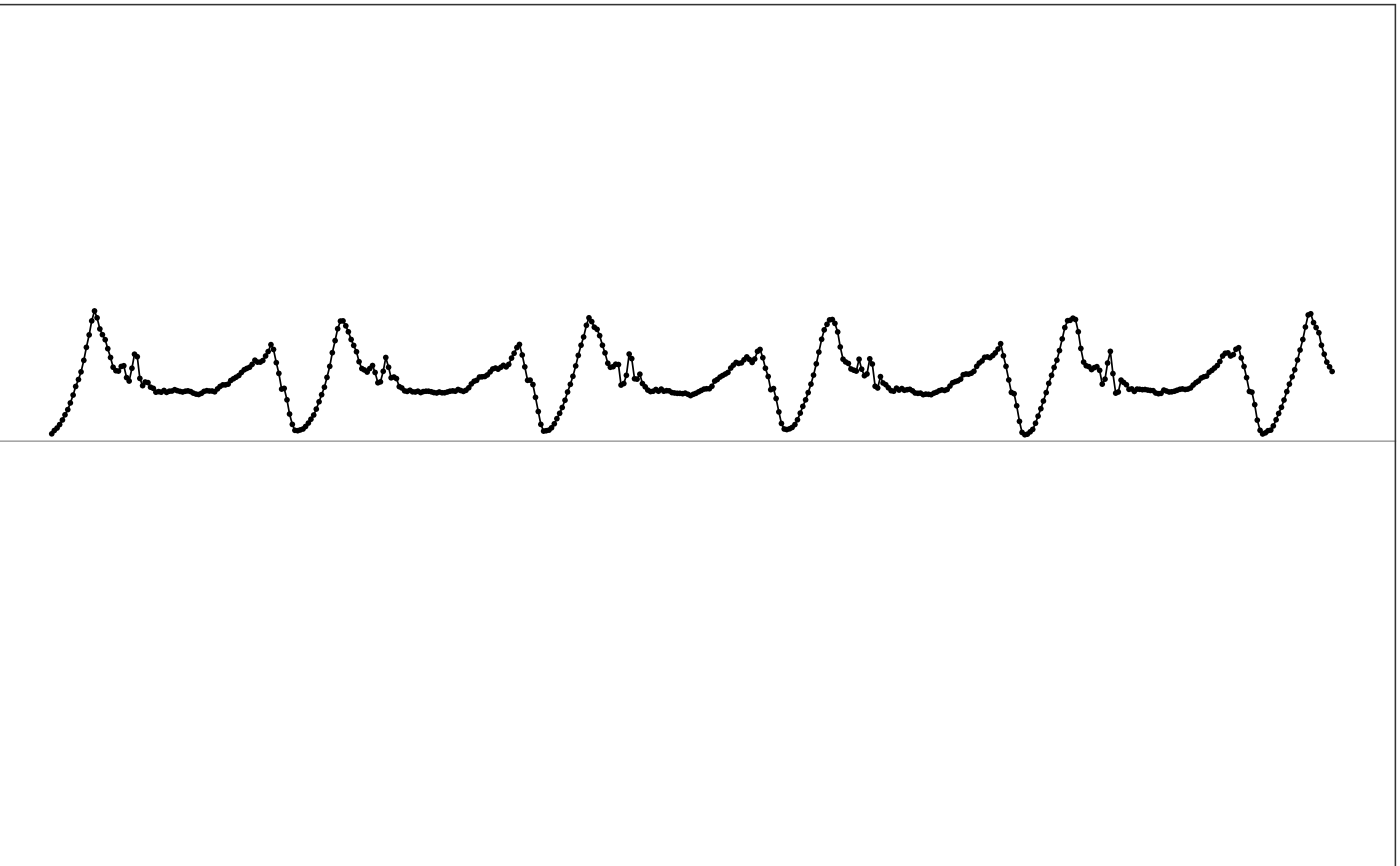bandlimited_interp function takes a multi-channel signal and applies a bandlimited interpolation filter to the signal to change its sampling rate.

bandlimited_interp(df, orig_sr, new_sr)

## Arguments

df dataframe. The input multi-channel signal. The first column is timestamps in POSXlct format. The rest columns are signal values. number. Sampling rate in Hz of the input signal. number. The desired sampling rate in Hz of the output signal.

## Value

dataframe. Filtered signal.

## Details

This function filters the input multi-channel signal by applying a bandlimited interpolation filter. See resample for the underlying implementation.

## How is it used in MIMS-unit algorithm?

This function is not used in the released version of MIMS-unit algorithm, but has once been considered to be used after extrapolation to harmonize sampling rate before filtering. But in the end, we decided to use linear interpolation before extrapolation to increase the sampling rate to 100Hz, so this method is no longer needed.

Other filtering functions: bessel(), iir(), remove_average()

## Examples

  # Use sample data
df = sample_raw_accel_data

# View input
illustrate_signal(df, plot_maxed_out_line = FALSE)# Apply filtering that uses the same setting as in MIMSunit algorithm
output = bandlimited_interp(df, orig_sr=80, new_sr=30)

# View output
illustrate_signal(output, plot_maxed_out_line = FALSE)